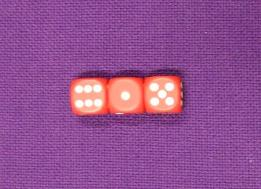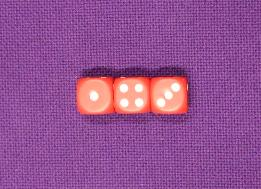#### You may also like### Pebbles

Place four pebbles on the sand in the form of a square. Keep adding as few pebbles as necessary to double the area. How many extra pebbles are added each time?### Bracelets

Investigate the different shaped bracelets you could make from 18 different spherical beads. How do they compare if you use 24 beads?### Sweets in a Box

How many different shaped boxes can you design for 36 sweets in one layer? Can you arrange the sweets so that no sweets of the same colour are next to each other in any direction?

# Three Dice

## Three Dice

Take a look at some ordinary dice.
What do you notice about the way the numbers are arranged?

Now look at these three dice in a row:The numbers on the tops of the dice read $6$, $1$ and $5$.

What do the numbers on the top add up to?

Can you use what you found out about the way the numbers are arranged to say what numbers are on the bottom of the dice? Were you correct?

What is the sum of the numbers on the bottoms of the dice?

Let's try that again.
This time the numbers on the top read $1$, $4$ and $3$.Can you work out the total?
Can you work out the numbers on the bottom and their total?

Try out some arrangements yourself. Each time record the sum of the numbers on the top and the sum of the numbers on the bottom.

Do you notice a relationship between the 'top sum' and the 'bottom sum'?
Can you explain it?

I experimented with arrangements where the top sum is a multiple of three, and find that in each case the bottom sum is also a multiple of three. Is it always true?

I try to arrange the dice so that the top and bottom sums are both multiples of four, but can't seem to be able to do it. Can you? Can you explain what you find out?

On the other hand, if I arrange four dice in a row it is easy to make the top and bottom sums both multiples of four. Can you arrange four dice so that the top and bottom sums are both multiples of three? Can you explain what you find out?

### Why do this problem?

This problem encourages children to explain observations and to generalise.  It requires a good understanding of multiplication.  It may also introduce the idea that opposite faces of a dice add to seven, if that is something with which learners are not already familiar.

### Possible approach

Before you introduce the problem give the children an opportunity to look at and then compare several dice in order to find the connection between opposite faces.

You could then introduce the problem with three large dice.  Ask children to calculate the sum of the top numbers and the sum of the bottom numbers, and record the results on the board.  You could try this a few times altogether, recording the sums each time. Ask the group to try more examples in pairs, then add these findings to the board.  Make sure  they check their calculations!  Gather the group together again and invite learners to comment on anything they notice. Challenge them to explain why the sum of the top and bottom faces added together is always $21$.  If they have not realised that opposite faces add to $7$, then you may have to  ask a few probing questions about a single dice.

Move on to introduce the second part of the problem, perhaps by arranging the dice in a particular way, for example with $1$, $2$ and $3$ on the top faces.  You could remark on the fact that both the top and bottom sums are multiples of $3$ and set the children off on investigating whether, if the top sum is a multiple of $3$, then the bottom sum is always a multiple of $3$ too.

After a suitable length of time, bring everyone together again to discuss their findings. This gives you a chance to talk about proof in mathematics.  The first stage for children is looking for counter examples to a theory.  Ask the group whether anyone has found an example when the top sum was a multiple of $3$ but the bottom sum wasn't.  Is that enough for us to say that it will ALWAYS be the case?  What if we just haven't found that one way of arranging the dice that gives us a multiple of $3$ on the top and a multiple of something else (not $3$) on the bottom?  The justification (or proof) might be difficult for some to articulate but remind learners what we know about the total sum of the top and bottom numbers (it is $21$).  What can they say about $21$?

You may like to pose the final part of the problem for learners to think about over a longer period of time and perhaps devote some space on your wall for them to contribute findings.

### Key questions

What do you notice about the totals of the top and bottom faces?
How can you explain your observation?
What do you know about opposite faces of a dice?
How do you know that will ALWAYS be the case?

### Possible extension

Encourage children to ask their own questions to investigate.  They could write up their conjectures and discoveries on your maths wall.

### Possible support

At least three dice per pair should be available along with miniwhiteboards or paper/books for recording. A multiplication square might be useful for the second part of the activity so that pairs can check multiples of $3$.